## LetsPlayMaths.Com

WELCOME TO THE WORLD OF MATHEMATICS

# Class 8 - Rhombus Worksheet 2

1. One side of a rhombus is 23 cm. Find the perimeter of the rhombus.

a) 56 cm                     b) 69 cm
c) 90 cm                     d) 92 cm

2. The two diagonals are not necessarily equal in a _____.

a) Square                     b) Rectangle
c) Rhombus                 d) None of these

3. When a parallelogram will be called rhombus?

a) All its sides are equal
b) All opposite angles are equal
c) Diagonals bisects each other
d) All of the above

4. A rhombus can be _____.

a) Square                     b) Rectangle
c) Trapezium                d) None of these

5. A rhombus is a parallelogram in which _____ sides are equal.

a) 2                     b) 3
c) 4                     d) None of these

6. If all sides of a quadrilateral are equal, then it is a _____.

a) Rectangle                    b) Square
c) Rhombus                     d) Both B & C

7. All rhombus are squares. Mark True / False.

a) True                     b) False

8. Diagonals of a rhombus are equal and perpendicular to each other. Mark True / False.

a) True                     b) False

9. Every square is a rhombus. Mark True / False.

a) True                     b) False

10. A rhombus can be constructed uniquely, if both diagonals are given. Mark True / False.

a) True                     b) False

11. Every rhombus is not a trapezium. Mark True / False.

a) True                     b) False

12. A rhombus is a regular quadrilateral. Mark True / False.

a) True                     b) False

13. If the adjacent sides of a parallelogram are equal, then the parallelogram is a _____.

a) Rectangle                    b) Trapezium
c) Rhombus                     d) Square

14. If diagonal of a quadrilateral bisects both the angles, then it is a _____.

a) Kite                       b) Parallelogram
c) Rectangle             d) Rhombus

15. ABCD is a rhombus, find the perimeter.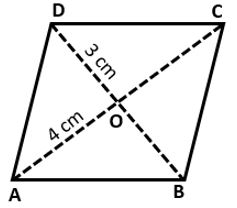a) 16 cm                      b) 12 cm
c) 20 cm                      d) 28 cm

16. ABCD is a rhombus, find the value of ∠CAD.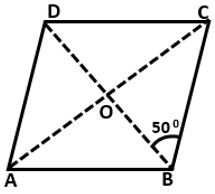a) 20°                      b) 30°
c) 35°                      d) 40°

17. ABCD is a rhombus, find the value of ∠ABC.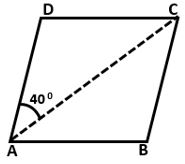a) 80°                      b) 100°
c) 90°                      d) 110°

18. ABCD is a rhombus, find the value of ∠ADC.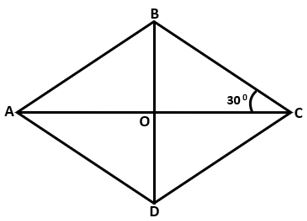a) 80°                      b) 120°
c) 90°                      d) 110°

19. In the below given figure, ABCD is a rhombus whose diagonals AC and BD intersect at a point O. If side AB is 10 cm and diagonal BD is equal to 16 cm. Find the length of AC.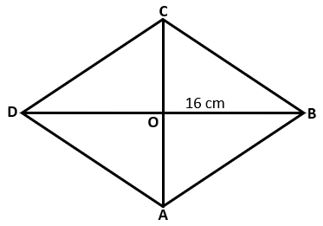a) 12 cm                      b) 16 cm
c) 10 cm                      d) 28 cm

20. Find the value of a, b and c in the below given rhombus.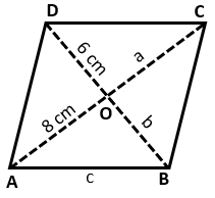a) a = 6 cm, b = 8 cm, c = 10 cm
b) a = 10 cm, b = 8 cm, c = 6 cm
c) a = 8 cm, b = 6 cm, c = 10 cm
d) a = 6 cm, b = 10 cm, c = 8 cm

Rhombus Worksheet - 1

Rhombus Worksheet - 2